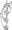# Fundamentals of HDL December 2010

Note: 1. Answer any FIVE full questions, selecting at least TWO questions from each part.

2. Missing data may be suitably assumed.

PART-A

1  a. Discuss the needs of HDL.

b.  With general syntax and suitable examples, explain the shift operators available in VHDL and verilog.

c.   Differentiate between an entity and a symbol.

2  a. What do you mean by the data flow style of description? Explain its features with a suitable example.

b. Write a data flow description VHDL for a system that has three 1 -bit inputs a(l), a(2) and a(3); and one 1-bit output b. The least significant bit is a(l); and b is 1, only when (a(l)a(2)a(3)) = 1, 3, 6 or 7 (all in decimal). Otherwise b is 0. Derive a minimized Boolean function of the system and write the data flow description.

C. With a suitable example, explain the concept of signal declaration.

3  a. With syntax of CASE statement in VHDL and verilog, discuss its facts,

b. Write a behavioral description of a 4-bit binary counter, in verilog.

4 a. Write a VHDL structural description for full adder, using two half adders.

b.  What is the advantage of structural coding in verilog, compared to structural coding in VHDL?

c.   Define state machine. Using the state machine concept, showing all the details, design a counter, which counts ^0, .2, 3, 5, 7 . Write the VHDL code for the same. (Use JK flip- flop).

PART-B

5 a.     With declaration syntax of procedure, explain its facts.

b.    Write a verilog function to find the largest of the two signed numbers.

c.    Bring out the differences between functions and procedures.

6  a. What do you understand by a file in HDL? List out the VHDL procedures for file processing.

b.  With syntax, explain the package and the package body.

c.   Write VHDL code for the state diagram shown in figure Q6 (c).7 a. Discuss the facts and limitations of mixed language description.

b.  With mixed language description of full adder, explain the invoking of VHDL entity from a verilog module.

8 a Define synthesis.

b. With a neat flow chart,, explain the steps involved in a synthesis process, .          (08 Marks)

c.   Draw the gate level synthesis information, extracted from the following verilog code.

d. Explain the mapping of the. signal assignment statement, y<=x; to gate level, with a suitable example.